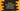# Python String isspace() explanation with example## What is isspace() :

isspace() is used to check if a string contains all white space characters or not. If your program can’t handle white spaces, this method really comes handy. For example, on your server, you can confirm a string before performing any operation on it.

In this tutorial, we will show you how to use isspace() with different examples.

## Syntax of isspace() :

The syntax of isspace() is as below :

``str.isspace()``

This is an inbuilt method and you don’t need anything to import. str is the string where you are checking for white space.

## Return value of isspace() :

isspace() returns a Boolean value. If all characters are whitespace, it will return True, else it will return False.

## Whitespace characters :

In python, following characters are considered as a whitespace character :

``````- ' ' : space
- '\n' : new line
- '\v' : vertical tab
- '\t' : horizontal tab
- '\f' : feed
- '\r' : carriage return``````

So if the string contains only any of these characters or a subset of these characters, isspace() will return True.

## Example python program :

Let’s learn isspace() with a simple example :

``````str1 = ""
str2 = "Hello"
str3 = "Hello "
str4 = " "
str5 = "\n"
str6 = "\v"
str7 = "\t"
str8 = "\f"
str9 = "\r"
str10 = "\n \r \f"

print("str1 : {}".format(str1.isspace()))
print("str2 : {}".format(str2.isspace()))
print("str3 : {}".format(str3.isspace()))
print("str4 : {}".format(str4.isspace()))
print("str5 : {}".format(str5.isspace()))
print("str6 : {}".format(str6.isspace()))
print("str7 : {}".format(str7.isspace()))
print("str8 : {}".format(str8.isspace()))
print("str9 : {}".format(str9.isspace()))
print("str10 : {}".format(str10.isspace()))``````

It will print the below output :

``````str1 : False
str2 : False
str3 : False
str4 : True
str5 : True
str6 : True
str7 : True
str8 : True
str9 : True
str10 : True``````

As you can see here, the first three strings give False.

• The first string is an empty string. It doesn’t have any whitespace.
• The second string is not empty but it doesn’t have any whitespace.
• The third string has one space but it also contains one word. isspace() returns True only if all are whitespace.